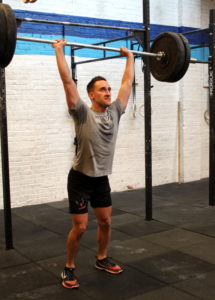## Monday, December 4, 2017

### Monday, December 4, 2017as many rounds as possible in 15 minutes:
10x pull-up
20x overhead walking lunge @ 50/35-lbs.
30x squatOutside The Box”
as many rounds as possible in 15 minutes:
10x *pull*
20x walking lunge
30x squat

Post score to comments.

### 1 Comment

1.–Scores–
Cristian D.: 4 rounds + 7x strict bodyrow (20-lbs.; sub: sa front rack lunge, 7x strict bodyrow, 2/3x squat)
Joya TD.: 4 rounds + 5x pull (10-lbs.; sub: 1/2x band, 2/3x squat)
Jen M.: 3 rounds + 16x lunge (20-lbs.; sub: 1/2x band, 2/3x squat)
Simon B.: 3 rounds + 5x pull (15-lbs.; sub: sa front rack lunge, 1/2x band, 2/3x squat)
Mead O.: 3 rounds (15-lbs.; sub: sa front rack lunge, 1/2x band, 2/3x squat)
Linda K.: 4 rounds + 7x lunge (10-lbs.; sub: sa front rack lunge, 7x strict bodyrow, 2/3x squat)
Borja G.: 5 rounds + 10x pull
Hari P.: 5 rounds + 4x pull (40-lbs.)
Daniel S.: 3 rounds + 19x lunge (15-lbs.; sub: 1/2x band)
Anna G.: 5 rounds + 1x pull (15-lbs.; sub: 1/2x band)
Sara R.: 4 rounds + 5x pull (sub: 1/2x band, step-up, 20″ 1/2x glute bridge + 1/2x double under)
Tyler B.: 4 rounds + 22x squat (40-lbs.; sub: 1/2x pull)
Kate H.: 4 rounds + 5x pull (25-lbs.; sub: 1/2x band)
Samuel B.: 4 rounds + 5x pull (20-lbs.; sub: sa front rack lunge, 1/2x band)
Elizabeth R.: 4 rounds + 11x lunge (15-lbs.; sub: 1/2x band)
Collin S.: 5 rounds + 3x lunge (30-lbs.; sub: 1/2x pull/sit-up)
Kevin K.: 4 rounds + 11x squat (35-lbs.; sub: 1/2x pull)
Jason O.: 4 rounds + 7x pull
Keith C.: 4 rounds + 13x lunge (20-lbs.; sub: 1/2x strict pull)
Charles B.: 3 rounds + 10x squat (30-lbs.)
Sean F.: 4 rounds + 2x lunge
Luke B.: 5 rounds + 11x lunge (35-lbs.; sub: 1/2x pull)
Giovanni M.: 6 rounds + 10x lunge (10-lbs.; sub; 1/2x band)
Walt C.: 5 rounds + 4x lunge (35-lbs.; sub: 1/2x pull)
Denny W.: 5 rounds + 10x lunge (35-lbs.)
Jamie Ac.: 5 rounds + 5x pull (10-lbs.; sub: 1/2x band)
Amy S.: 5 rounds + 5x lunge
Jin D.: 5 rounds + 3x pull (25-lbs.; sub: 1/2x band)
Thomas R.: 5 rounds + 4x pull (40-lbs.)
Aaron B.: 5 rounds + 8x lunge (25/15-lbs.; sub: 1/2x band)
Jason S.: 6 rounds + 8x squat (35-lbs; sub: 1/2x band)
David C.: 4 rounds + 8x lunge (35-lbs.)
Christopher J.: 5 rounds (30-lbs.; sub: 1/2x pull)
Jason G.: 4 rounds + 20x squat (25-lbs.; sub: 1/2x band)
Maria L.: 4 rounds + 20x lunge (15-lbs.; sub: 1/2x band)
Cassie S.: 5 rounds (25-lbs.; sub: 1/2x pull)
RJ A.: 4 rounds + 5x pull (20-lbs.; sub: 1/2x strict pull)
Seth C.: 4 rounds + 15x squat (20-lbs.; sub: strict bodyrow)
Diana G.: 5 rounds + 10x squat (10-lbs.; sub: 1/2x pull)
Chase H.: 6 rounds + 5x pull (35-lbs.)
Alex M.: 6 rounds + 2x pull (40-lbs.)
Dave K.: 5 rounds (35-lbs.)
Dave R.: 6 rounds + 8x pull
Yordano L.: 6 rounds (25-lbs.; sub: 1/2x pull)
Lisa C.: 6 rounds + 1x pull (25-lbs.; sub: sa front rack lunge)
Francisco L.: 5 rounds (35-lbs.)
Donal O.: 4 rounds + 9x pull (35-lbs.; sub: squat/step-up)
David Sc.: 6 rounds + 2x pull (30-lbs.)
Diana D.: 6 rounds (15-lbs.; sub: sa front rack lunge, 1/2x band, 2/3x squat)
Joe T.: 6 rounds + 4x squat (35-lbs.)
Brad G.: 6 rounds (30-lbs.)
Ralph A.: 4 rounds + 5x pull (25-lbs.; sub: 1/2x pull)
Ross B.: 6 rounds + 3x squat (35-lbs.; sub: 1/2x pull)
Eduardo S.: 4 rounds + 5x pull-up (30-lb. db)
Michael H.: 5 rounds + 6x pull-up
Chris H.: 6 rounds + 10x lunge
Ralph B.: 4 rounds + 8x lunge
Josh M.: 4 rounds + 7x squat (35-lb. db; sub: single-arm step-up, 20″)
Charlie K.: 4 rounds + 10x pull-up (5 rounds)
Anna Go.: 4 rounds + 10x bodyrow (15-lb. db; sub: strict bodyrow)
Mark Ab.: 5 rounds + 8x pull-up (35-lb. db)
Megan K.: 4 rounds (30-lbs.; sub: 7/10x band pull-up)
Sergio L.: 3 rounds (25-lbs.; sub: band pull-up)
Elise C.: 6 rounds + 1 squat (sub: 1/2x pull-up)
Stephen K.: 4 rounds + 10x lunge (45-lbs.)
Max M.: 5 rounds + 9x pull-up (45-lbs.)
Holmes H.: 4 rounds + 14x lunge (20-lbs; sub: band pull-up)
Brian K.: 3 rounds + 7x squat (35-lbs.; sub: 1/2x strict pull-up)
Sean Mc.: 5 rounds + 13x squat
Sean Q.: 4 rounds + 20x lunge
Travers O.: 6 rounds + 4x pull-up (25-lbs.; sub: 1/2x spu, sa fs)
Jesse M.: 4 rounds + 1x pull up (30-lbs; sub: band pull-up)
Candida M.: 4 rounds + 4x squat (25-lbs.)
Miranda B.: 4 rounds + 2x lunge (30-lbsx; sub: 1/2x band pull-up)
Andrew Z.: 5 rounds + 8x lunge (45-lbs.)
Chris C.: 6 rounds + 10x pull-up
Rachel B.: 4 rounds + 7 pull-ups (sub: 7/10x band pull-up)
Kat S.: 4 rounds + 4x pull-up (sub: 7/10x band pull-up)
Katherine C.: 3 rounds + 20x lunge (10-lbs.; sub: band pull-up)
Adrienne V.: 4 rounds + 6x pull-up (25-lbs.; sub: band pull-up)
Mark Co.: 4 rounds + 7 lunge
Bill G.: 5 rounds + 9x lunge (sub: 7/10x strict pull-up)
Krishnan S.: 4 rounds + 10x pull-up (35-lbs.)
Christian M.: 5 rounds (40-lbs.)
Patrick J.: 4 rounds + 10x pull-up (40-lbs.)